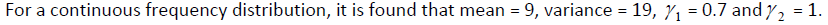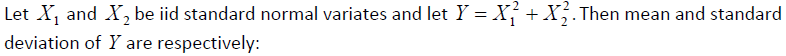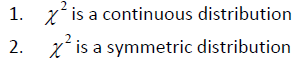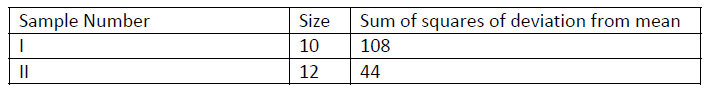### Solved Question Paper for Statistical Investigator Grade - I & Asstt. Director of Census Operations (T) in the Office of RGI conducted by UPSC

11. The underlying assumptions for the application of student’s t‐test for the difference of means are:

1. The two samples are random and independent of each other.
2. Population variances are equal and known.
3. Parent population from which the samples have been drawn is uniform.

Which of the above statements is/are correct?
(a) 1 only
(b) 2 only
(c) 3 only
(d) 1, 2 and 3

12. Consider the following statements for a frequency distribution:
1. Mean, Mean deviation and standard deviation have the same unit.
2. HM ≤ GM ≤ AM

Which of the above statements is/are correct?
(a) 1 only
(b) 2 only
(c) Both 1 and 2
(d) Neither 1 nor 2

13. For a continuous frequency distribution, it was found that mean = 50, mode = 30 and variance = 16.

Consider the following statements:
Statement‐1: The larger tail of the distribution lies towards the higher values of the variate.
Statement‐2: Karl Pearson’s coefficient of skewness is positive.

Which one of the following is true in respect of the above statements?
(a) Both Statement‐1 and Statement‐2 are correct and Statement‐2 is the correct explanation of Statement‐1
(b) Both Statement‐1 and Statement‐2 are correct and Statement‐2 is NOT the correct
explanation of Statement‐1
(c) Statement‐1 is correct but Statement‐2 is NOT correct
(d) Statement‐1 is NOT correct but Statement‐2 is correct

14. Consider the following data:
x: 5, 4, 3, 2, 1
y: 1, 2, 3, 4, 5

What is the rank correlation coefficient between x and y for the above data?
(a) 1
(b) ‐1
(c) 0.5
(d) ‐0.5

15. The underlying assumptions for the application of student’s t‐test for single mean are:
Assumption‐1: The parent population from which the sample is drawn is normal
Assumption‐2: The sample observations are independent
Assumption‐3: The population standard deviation σ is known

Which of the above assumptions are true?
(a) 1 and 2 only
(b) 2 and 3 only
(c) 1 and 3 only
(d) 1, 2 and 3

16.The nature of the frequency distribution is:
(a) Symmetric and platykurtic
(b) Symmetric and leptokurtic
(c) Negatively skewed and platykurtic
(d) Positively skewed and leptokurtic

17.(a) 1 and 2
(b) 2 and 2
(c) 2 and 4
(d) 4 and 4

18. Consider the following statements:Which of the above is/are correct?
(a) 1 only
(b) 2 only
(c) Both 1 and 2
(d) Neither 1 nor 2

19. Means of two series are m and n. Let each observation of first series be increased by x and each observation of the second series be increased by y. New means of the two series now become p and q. Then consider the following statements:
1. p : q = m : n
2. p + q = m + n + x + y
3. p – q = m – n
4. (p – x)(q – y) = mn

Which of the above are correct?
(a) 1 and 2
(b) 1 and 3
(c) 2 and 4
(d) 2 and 3

20. Two random samples gave the following results:To test the hypothesis of equality of population variances, the value of test statistic F is:
(a) 3
(b) 0.5
(c) 4
(d) 0.25

Share:

## Featured Post

### UPSC Civil Service Preliminary Paper-1 Previous Year Solved Question Papers

Civil Service Preliminary Paper-1 Previous Year Solved Questions for the year 2019 Civil Service Preliminary Paper-1 Previous Year Solved Qu...Name

Email *

Message *